# 有压管道内关阀水锤的三维模拟研究A Three-Dimensional Simulation about Water Hammer Caused by Valve Closure in Pressure Pipeline

• 全文下载: PDF(903KB)    PP.136-144   DOI: 10.12677/MOS.2018.73017
• 下载量: 530  浏览量: 1,295   科研立项经费支持

In this paper we study the case that ball is closing in a pressure pipeline. The main research method is computational fluid dynamics software; here we introduce the source term: the compressibility of water. The target of building model and simulation for three-dimensional water hammer are accomplished. Experimental results are used to verify that the three-dimensional model can simulate the pressure of water hammer accurately when valve is closing. Compared with one-dimensional model, three-dimensional model is more precise, and the three-dimensional flow fluid during the direct water hammer can also be demonstrated vividly. The shape of valve and the valve closure time are also studied. On the basis of three-dimensional model, we can simulate the water hammer more accurately and precisely. The results have important theoretical value and practical engineering value.

1. 研究背景

2. 物理模型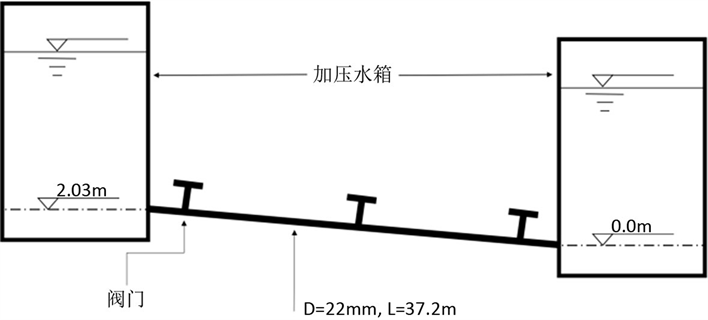Figure 1. Schematic diagram of experimental set-up

3. 数学模型

3.1. 控制方程

1) 三维动量和连续性方程

$\frac{\partial \rho }{\partial t}+\frac{\partial \rho {u}_{x}}{\partial x}+\frac{\partial \rho {u}_{y}}{\partial y}+\frac{\partial \rho {u}_{z}}{\partial z}={S}_{m}$ (1)

$\frac{\partial }{\partial t}\left(\rho {u}_{i}\right)+\frac{\partial }{\partial {x}_{i}}\left(\rho {u}_{i}{u}_{j}\right)=-\frac{\partial P}{\partial {x}_{i}}+\frac{\partial }{\partial {x}_{i}}\mu \left(\frac{\partial {u}_{i}}{\partial {x}_{j}}+\frac{\partial {u}_{j}}{\partial {x}_{i}}\right)+\rho {g}_{i}+{F}_{i}$ (2)

2) 湍流模型

$\frac{\partial }{\partial t}\left(\rho k\right)+\frac{\partial }{\partial {x}_{i}}\left(\rho k{u}_{i}\right)=\frac{\partial }{\partial {x}_{i}}\left({\Gamma }_{k}\frac{\partial k}{\partial {x}_{i}}\right)+{G}_{k}-{Y}_{k}+{S}_{k}$ (3)

$\frac{\partial }{\partial t}\left(\rho \omega \right)+\frac{\partial }{\partial {x}_{i}}\left(\rho \omega {u}_{i}\right)=\frac{\partial }{\partial {x}_{j}}\left({\Gamma }_{\omega }\frac{\partial \omega }{\partial {x}_{j}}\right)+{G}_{\omega }+{Y}_{\omega }+{D}_{\omega }+{S}_{k}$ (4)

3) 液体状态方程

${\rho }_{l}={\rho }_{l,0}{\left(\frac{K}{{{K}^{\prime }}_{l,0}}\right)}^{1/n}$ (5)

$K={{K}^{\prime }}_{l,0}+n\Delta {p}_{l}^{*}$ (6)

$\Delta {p}_{l}^{*}={p}_{l}^{*}-{\rho }_{l,0}^{*}$ (7)

3.2. 模型求解

3.3. 几何模型和边界条件

4. 三维计算结果

4.1. 模型验证

4.2. 阀门形状对直接水锤的影响

4.3. 阀门关闭时间对直接水锤的影响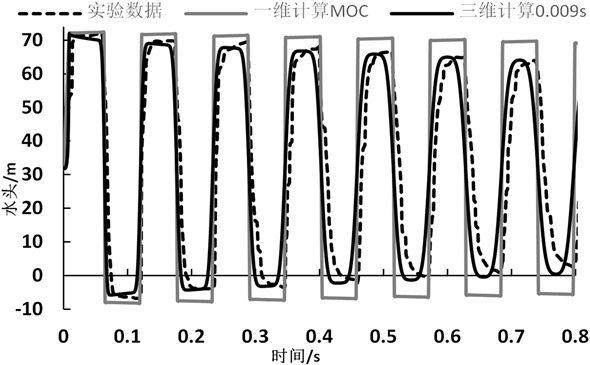Figure 2. Comparative analysis of 3D, 1D and experiment(a)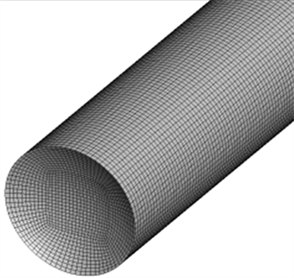(b)

Figure 3. Schematic plots for different shape of valve: (a) Plane; (b) Sphere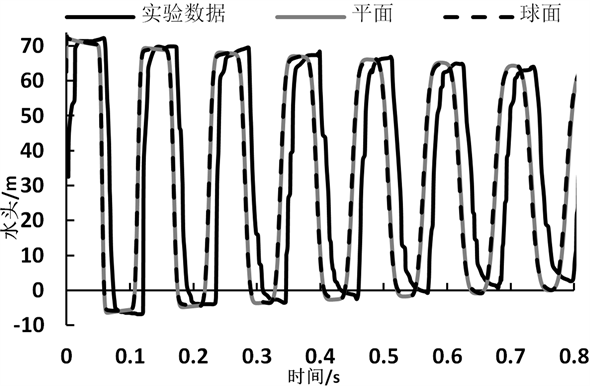Figure 4. Comparative analysis of the results between different shape of valve and experiment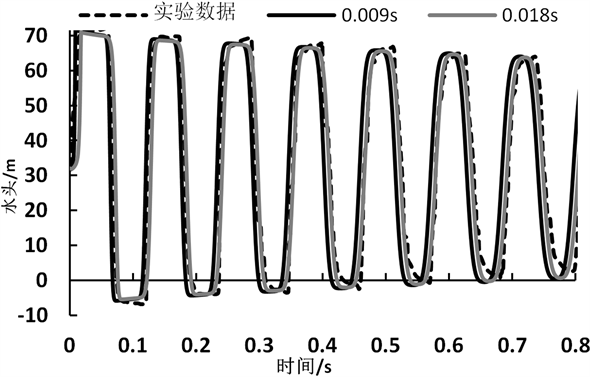Figure 5. Comparative analysis of the results between different closing time and experiment

1) 0 s关闭的球阀是一组附加的参考对比值，采用0 s关阀相对于采用盲端作为对比更具有代表性，也可以排除一些不必要的干扰，从而使对比具有更高的可信度。

2) 0.009 s的球阀关闭时间是实验中采用的关阀时间，这种关阀时间在最大程度上保持了与实验的一致性，这组数值模拟对实验的还原度最高，所以具有很高的参考价值。

3) 0.018 s的球阀关闭时间也是一组附加的参考对比值，因为之前的数值模拟数据和实验数据的对比过程中，发现数值模拟数据在时间上总是与实验数据有较大差异，这种差异有两点很明显的表现：第一点是水锤波的产生时间比实验测得要早，第二点是水锤波的周期2 L/a会随着时间增长。所以我们尝试将关阀时间延长，以期得到与实验数据比较接近的数值模拟结果。

$2L/a=2×37.2÷1319\approx 0.05641\text{s}$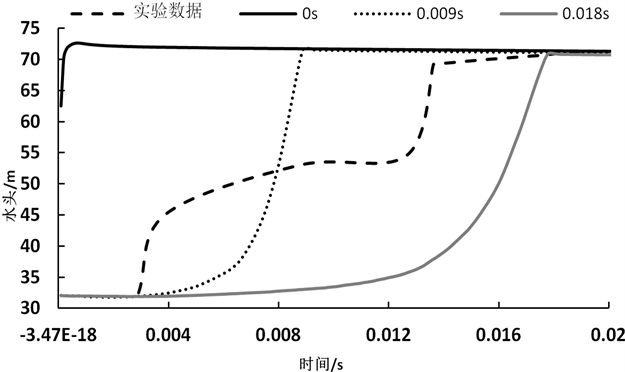Figure 6. Magnification of Figure 5, part one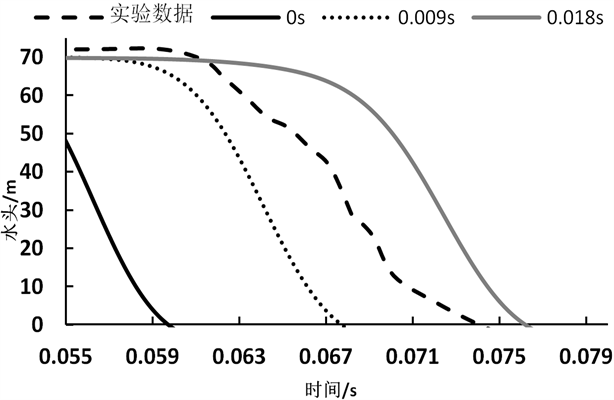Figure 7. Magnification of Figure 5, part two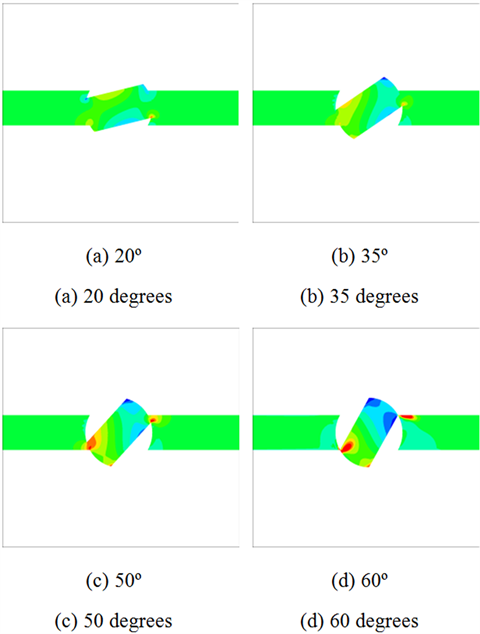Figure 8. Velocity field of fluid during the ball valve is closing

4.4. 三维流场

5. 结论

  黄毅, 陈锋. 长距离输水工程末端关阀方式对水锤防护的影响[J]. 人民长江, 2018, 49(5): 70-73.  马世波, 张健. 长距离输水工程停泵水锤防护措施研究[J]. 人民长江, 2009, 40(1): 85-87.  刘发明. 给水管路系统水锤数值计算及管路结构响应研究[D]: [硕士学位论文]. 武汉: 武汉理工大学, 2014.  毕延龄. 输水系统的水锤及水锤防护[J]. 建筑技术通讯, 1988(2): 30-34.  李健, 徐广明. 水锤的危害与防范[J]. 中国科技信息, 2008(15): 49-50.  朱旭东. 湍流模型的比较、改进和应用[D]: [硕士学位论文]. 南京: 南京航空航天大学, 2005.  郭艳惠, 刘德友, 周领. 关阀过程中管内瞬变流一二维数值模拟对比[J]. 人民黄河, 2013, 35(9): 122-125.  杨帅. 基于MOC-CFD耦合方法的泵送系统瞬态特性研究[D]: [博士学位论文]. 杭州: 浙江大学, 2015.  王小庆, 金先龙, 曹源. 大规模输水隧道水锤效应三维数值模拟[J]. 上海交通大学学报, 2016, 50(1): 98-102.  宋汉戈, 刘世光. 三维流场可视化综述[J]. 系统仿真学报, 2016, 28(9): 1929-1936.  范晓丹, 刘韩生. 非恒定摩阻的TVD格式数值模拟水击衰减研究[J]. 水利水电学报, 2017, 36(3): 55-62.  张大帅. 非恒定摩阻及阀门关闭规律对水锤波的影响[D]: [硕士学位论文]. 西安: 西安理工大学, 2016.  邵杰, 李晓花, 郭振江, 等. 不同湍流模型在管道流动数值模拟中的适用性研究[J]. 化工设备与管道, 2016, 53(4): 66-71.  马跃先, 周琼, 李世英. 直接水击公式研究[J]. 人民黄河, 2017, 29(11): 80-81.  刘晶. 结构与非结构网格的生成、转化及应用[D]: [硕士学位论文]. 南京: 南京理工大学, 2006.